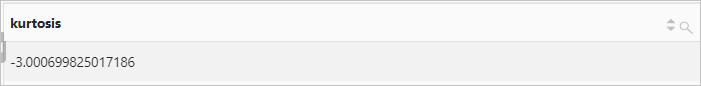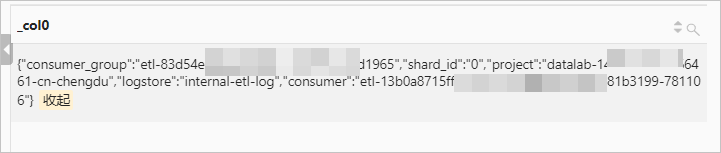arbitrary函数 arbitrary(x) 返回x中任意一个非空的值。
avg函数 avg(x) 计算x的算术平均值。
bitwise_and_agg函数 bitwise_and_agg(x) 返回x中所有值按位与运算（AND）的结果。
bitwise_or_agg函数 bitwise_or_agg(x) 返回x中所有值按位或运算（OR）的结果。
bool_and函数 bool_and(boolean expression) 判断是否所有日志都满足条件。如果是，则返回true。

bool_and函数等同于every函数。

bool_or函数 bool_or(boolean expression) 判断是否存在日志满足条件。如果存在，则返回true。
checksum函数 checksum(x) 计算x的校验和。
count函数 count(*) 统计所有的日志条数。
count(1) 统计所有的日志条数，等同于count(*)。
count(x) 统计x中值不为NULL的日志条数。
count_if函数 count_if(boolean expression) 统计满足指定条件的日志条数。
every函数 every(boolean expression) 判断是否所有日志都满足条件。如果是，则返回true。

every函数等同于bool_and函数。

geometric_mean函数 geometric_mean(x) 计算x的几何平均数。
kurtosis函数 kurtosis(x) 计算x的峰度。
map_union函数 map_union(x) 返回一列Map数据的并集。 如果Map中存在相同的键，则返回的键值为其中任意一个键的值。
max函数 max(x) 查询x中的最大值。
max(x, n) 查询x中最大的n个值。返回结果为数组。
max_by函数 max_by(x, y) 查询y为最大值时对应的x值。
max_by(x, y, n) 查询最大的ny值对应的x值，返回结果为数组。
min函数 min(x) 查询x中最小值。
min(x, n) 查询x中最小的n个值。返回结果为数组。
min_by函数 min_by(x, y) 查询y为最小值时对应的x值。
min_by(x, y, n) 查询最小的ny值对应的x值。返回结果为数组。
skewness函数 skewness(x) 计算x的偏度。
sum函数 sum(x) 计算x的总值。

## arbitrary函数

arbitrary函数用于返回x中任意一个非空的值。

### 语法

``arbitrary(x)``

x 参数值为任意数据类型。

### 示例

• 查询和分析语句
``* | SELECT arbitrary(request_method) AS request_method``
• 查询和分析结果## avg函数

avg函数用于计算x的算术平均值。

### 语法

``avg(x)``

### 参数说明

x 参数值为double、bigint、decimal或real类型。

double类型。

### 示例

• 查询和分析语句
``method: PostLogstoreLogs | SELECT avg(latency) AS avg_latency, Project GROUP BY Project HAVING avg_latency > 1000``
• 查询和分析结果## bitwise_and_agg函数

bitwise_and_agg函数用于返回x中所有值按位与运算（AND）的结果。

### 语法

``bitwise_and_agg(x)``

x 参数值为bigint类型。

bigint类型（二进制形式）。

### 示例

request_time字段的所有值进行按位与运算。

• 查询和分析语句
``* | SELECT bitwise_and_agg(status)``
• 查询和分析结果## bitwise_or_agg函数

bitwise_or_agg函数用于返回x中所有值按位或运算（OR）的结果。

### 语法

``bitwise_or_agg(x)``

x 参数值为bigint类型。

bigint类型（二进制形式）。

### 示例

request_time字段的所有值进行按位或运算。
• 查询和分析语句
``* | SELECT bitwise_or_agg(request_length)``
• 查询和分析结果## bool_and函数

bool_and函数用于判断是否所有日志都满足条件。如果是，则返回true。bool_and函数等同于every函数。

### 语法

``bool_and(boolean expression)``

### 参数说明

boolean expression 参数值为布尔表达式。

boolean类型。

### 示例

• 查询和分析语句
``* | SELECT bool_and(request_time < 100)``
• 查询和分析结果## bool_or函数

bool_or函数用于判断是否存在日志满足条件。如果存在，则返回true。

### 语法

``bool_or(boolean expression)``

### 参数说明

boolean expression 参数值为布尔表达式。

boolean类型。

### 示例

• 查询和分析语句
``* | SELECT bool_or(request_time < 20)``
• 查询和分析结果## checksum函数

checksum函数用于计算x的校验和。

### 语法

``checksum(x)``

x 参数值为任意数据类型。

### 返回值类型

string类型（BASE 64编码）。

### 示例

• 查询和分析语句
``* | SELECT checksum(request_method) AS request_method``
• 查询和分析结果## count函数

count函数用于计数。

### 语法

• 统计所有的日志条数。
``count(*)``
• 统计所有的日志条数。等同于`count(*)`
``count(1)``
• 统计x中值不为NULL的日志条数。
``count(x)``

x 参数值为任意数据类型。

integer类型。

### 示例

• 示例1：统计网站访问量。
• 查询和分析语句
``* | SELECT count(*) AS PV``
• 查询和分析结果• 示例2：统计包含request_method字段且字段值不为NULL的日志条数。
• 查询和分析语句
``* | SELECT count(request_method) AS count``
• 查询和分析结果## count_if函数

count_if函数用于统计满足指定条件的日志条数。

### 语法

``count_if(boolean expression)``

### 参数说明

boolean expression 参数值为布尔表达式。

integer类型。

### 示例

• 查询和分析语句
``* | SELECT count_if(request_uri like '%file-0') AS count``
• 查询和分析结果## geometric_mean函数

geometric_mean函数用于计算x的几何平均数。

### 语法

``geometric_mean(x)``

### 参数说明

x 参数值为double、bigint或real类型。

double类型。

### 示例

• 查询和分析语句
``* | SELECT geometric_mean(request_time) AS time``
• 查询和分析结果## every函数

every函数用于判断是否所有日志都满足条件。如果是，则返回true。every函数等同于bool_and函数。

### 语法

``every(boolean expression)``

### 参数说明

boolean expression 参数值为布尔表达式。

boolean类型。

### 示例

• 查询和分析语句
``* | SELECT every(request_time < 100)``
• 查询和分析结果## kurtosis函数

kurtosis函数用于计算x的峰度。

### 语法

``kurtosis(x)``

### 参数说明

x 参数值为double、bigint类型。

double类型。

### 示例

• 查询和分析语句
``*| SELECT kurtosis(request_time)``
• 查询和分析结果## map_union函数

map_union函数用于返回一列Map数据的并集。 如果Map中存在相同的键，则返回的键值为其中任意一个键的值。

### 语法

``map_union(x)``

x 参数值为map类型。

map类型。

### 示例

etl_context字段的值（map类型）聚合后，随机返回其中一个值（map类型）。

• 字段样例
``````etl_context: {
project:"datalab-148****6461-cn-chengdu"
logstore:"internal-etl-log"
consumer_group:"etl-83****4d1965"
consumer:"etl-b2d40ed****c8d6-291294"
shard_id:"0" }``````
• 查询和分析语句
``* | SELECT map_union(try_cast(json_parse(etl_context) AS map(varchar,varchar)))``
• 查询和分析结果## max函数

max函数用于查询x中最大的值。

### 语法

• 查询x中最大的值。
``max(x)``
• 查询x中最大的n个值，返回结果为数组。
``max(x, n)``

x 参数值为任意数据类型。
n 参数值为正整数。

### 示例

• 示例1：查询请求时长的最大值。
• 查询和分析语句
``* | SELECT max(request_time) AS max_request_time``
• 查询和分析结果• 示例2：查询请求时长的top 10。
• 查询和分析语句
``* | SELECT max(request_time,10) AS "top 10"``
• 查询和分析结果## max_by函数

max_by函数支持如下两种用法。

### 语法

• 查询y为最大值时对应的x值。
``max_by(x, y)``
• 查询最大的ny值对应的x值，返回结果为数组。
``max_by(x, y, n)``

x 参数值为任意数据类型。
y 参数值为任意数据类型。
n 大于0的整数。

### 示例

• 示例1：统计最高消费订单对应的时间点。
• 查询和分析语句
``* | SELECT max_by(UsageEndTime, PretaxAmount) as time``
• 查询和分析结果• 示例2：统计请求时长最大的3个请求对应的请求方法。
• 查询和分析语句
``* | SELECT max_by(request_method,request_time,3) AS method``
• 查询和分析结果## min函数

min函数用于查询x中最小值。

### 语法

• 查询x中最小值。
``min(x)``
• 查询x中最小的n个值，返回结果为数组。
``min(x,n)``

x 参数值为任意数据类型。
n 参数值为正整数。

### 示例

• 示例1：查询请求时长的最小值。
• 查询与分析语句
``* | SELECT min(request_time) AS min_request_time``
• 查询和分析结果• 示例2：查询请求时长最小的10个值。
• 查询和分析语句
``* | SELECT min(request_time,10)``
• 查询和分析结果## min_by函数

min_by函数支持如下两种用法。

### 语法

• 查询y为最小值时对应的x值。
``min_by(x, y)``
• 查询最小的ny值对应的x值。返回结果为数组。
``min_by(x, y, n)``

x 参数值为任意数据类型。
y 参数值为任意数据类型。
n 大于0的整数。

### 示例

• 示例1：返回最小请求时长的请求对应的请求方法。
• 查询和分析语句
``* | SELECT min_by(request_method,request_time) AS method``
• 查询和分析结果• 示例2：返回请求时长最小的3个请求对应的请求方法。
• 查询和分析语句
``* | SELECT min_by(request_method,request_time,3) AS time``
• 查询和分析结果## skewness函数

skewness函数用于计算x的偏度。

### 语法

``skewness(x)``

### 参数说明

x 参数值为double、bigint类型。

double类型。

### 示例

• 查询和分析语句
``*| SELECT skewness(request_time) AS skewness``
• 查询和分析结果## sum函数

sum函数用于计算x的总值。

### 语法

``sum(x)``

### 参数说明

x 参数值为double、bigint、decimal或real类型。

### 示例

• 查询和分析语句
``* | SELECT date_trunc('day',__time__) AS time, sum(body_bytes_sent) AS body_bytes_sent GROUP BY time ORDER BY time``
• 查询和分析结果